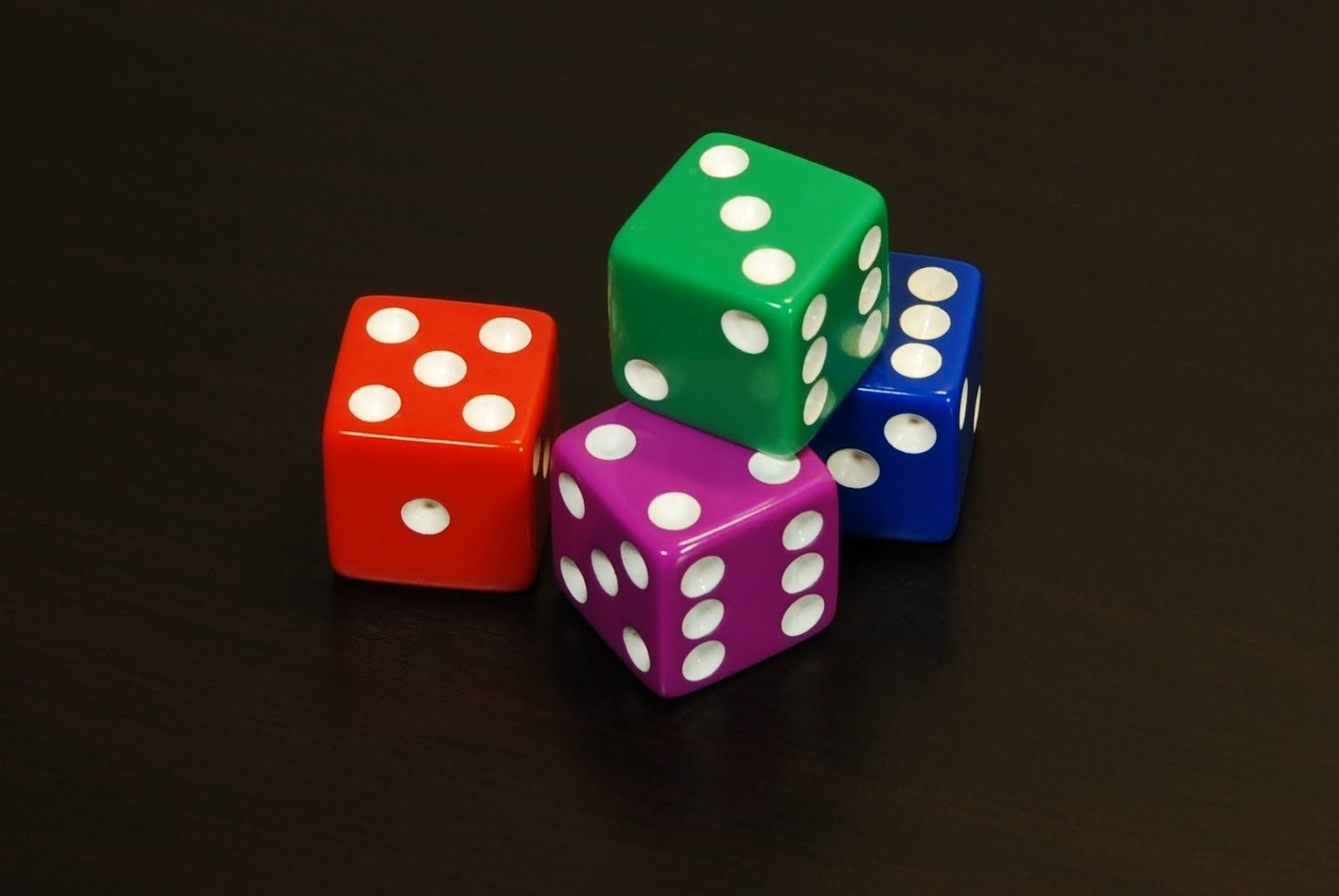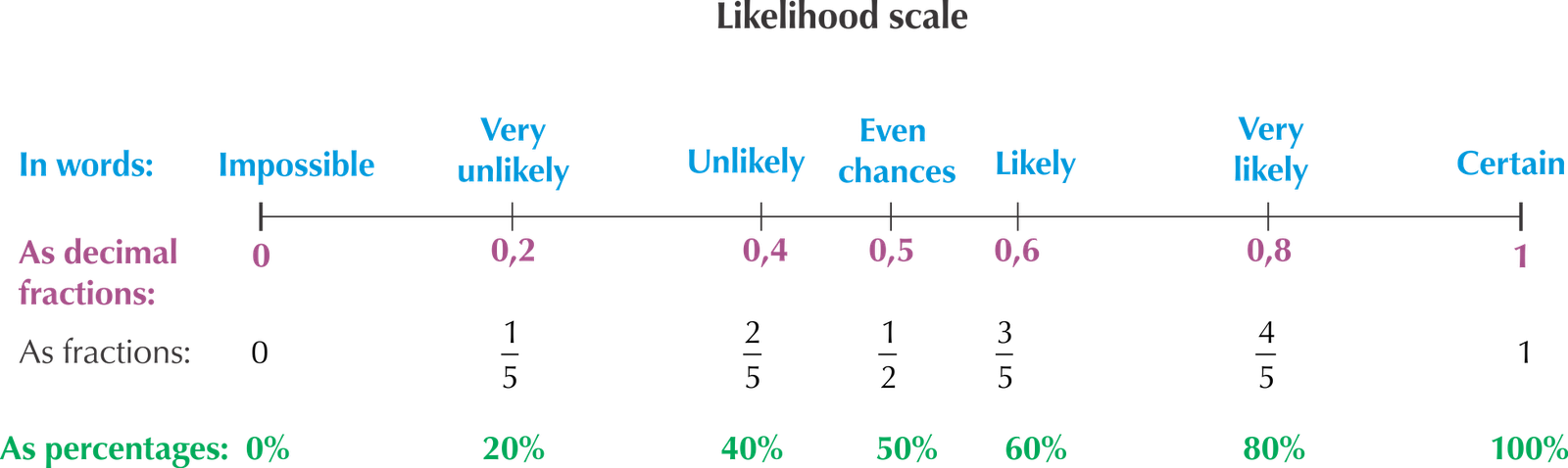Home Practice
For learners and parents For teachers and schools
Textbooks
Full catalogue
Pricing SupportLog in

We think you are located in United States. Is this correct?

# Chapter 7: Probability

## 7.1 Introduction and key concepts (EMG4Y)

Probability is the study of the chances of things happening in future. We often make statements like “I am sure it will never happen”; “I have no chance of winning the prize!”, “I am sure it is going to snow this year.” Each of the statements tries to predict a future event.

In this chapter we will learn about:

• the probabilty scale and making predicitions.
• games of chance and fair and unfair games.
• the difference between an event and an outcome.
• tree diagrams and two-way tables.
• weather predictions.### The probability scale (EMG4Z)

To describe the probability that something will happen we can use a probability scale. This scale is a continuous line that starts with impossible events at the left-hand end and ends with certain events at the right-hand end. All probabilities must fall somewhere on this line. An event that is described as “impossible” is one that we know will never happen, such as having 8 days in one week. An event is described as “certain” if we know that it will definitely happen, such as having a Monday in a week. Between the two ends of the line are word descriptions: very unlikely, unlikely, even chance, likely, very likely.

We can also give numbers to the probabilities on this scale. Remember, though, that each word description covers a range of continuous probabilities on the scale, and it doesn't match a particular number exactly. The word descriptions used describe the mathematical meaning.## Worked example 1: Working with the probability scale

Give each of these events a word description from the probability scale.

1. What are the chances of winning the Lottery if you buy a ticket every week for two months?
2. How likely is it that you will pass Maths Literacy this year?
3. What are the chances of rainfall in your area today?
4. What are the chances of a pregnant woman having a male child?
1. It is highly unlikely that a particular person will win the Lottery.
3. You can give your own answer, depending on the current weather and forecasts.
4. A $$\text{50}\%$$ (equal) chance.

Probabilities between $$\text{0}$$ and $$\text{1}$$ can be written as decimals, common fractions or percentages. So our probability scale could look like this:## Becoming familiar with the probability scale

Exercise 7.1

Work in a group to answer the following questions about the probability scale:

Think about five events that have different chances of happening.

Learners should come up with their own meaningful descriptions here.

Draw a probability scale with all the word labels written on it.

Discuss where these events should go on the probability scale and come to an agreement in your group. You might find that different people have different ideas about what the words on the scale mean.

It is useful for learners to have a discussion about this to help them see that the descriptions are somewhat subjective.

Write your five events on this probability scale.

Place these events on your probability scale:

A one in ten chance of pulling a red T-shirt out of your cupboard without looking.

At $$\text{0,1}$$ on the scale.

An $$\text{80}\%$$ chance of rain.

At $$\text{0,8}$$ on the scale.

A $$\frac{\text{1}}{\text{20}}$$ chance of having twins.

At $$\text{0,05}$$ (unlikely) on the scale.

A one in a million chance of being struck by lightning

Close to zero on the scale.

Write these probabilities as decimals and as percentages:

$$\frac{\text{1}}{\text{4}}$$

$$\text{0,25}$$; $$\text{25}\%$$

$$\frac{\text{4}}{\text{5}}$$

$$\text{0,8}$$; $$\text{80}\%$$

$$\frac{\text{1}}{\text{20}}$$

$$\text{0,05}$$; $$\text{5}\%$$

$$\frac{\text{3}}{\text{100}}$$

$$\text{0,03}$$; $$\text{3}\%$$

$$\frac{\text{6}}{\text{7}}$$

$$\text{0,86}$$; $$\text{86}\%$$

Discuss whether you think it is easier to describe a probability using a number or a word description.

Learner-dependent answer. Encourage learners to discuss the fact that will probably give different answers if they use words, while using a number ensures that everyone has the same understanding.